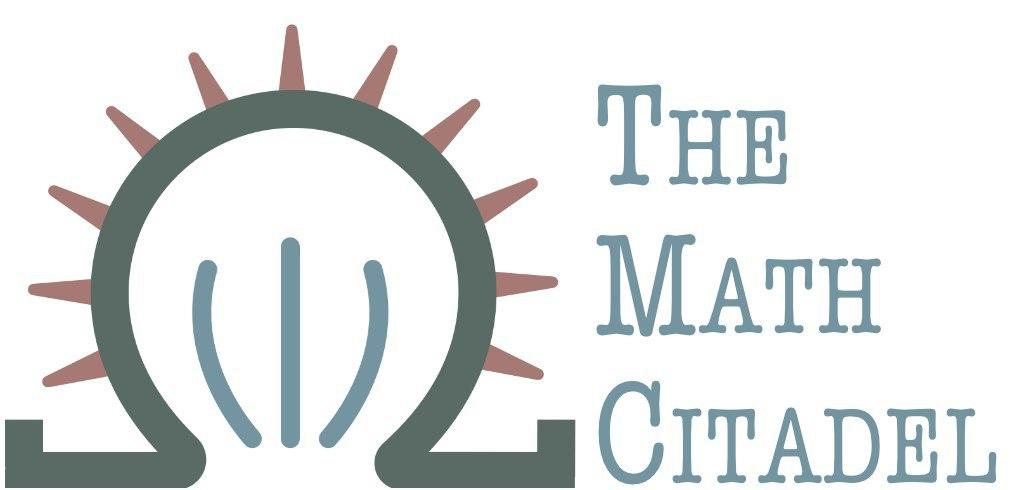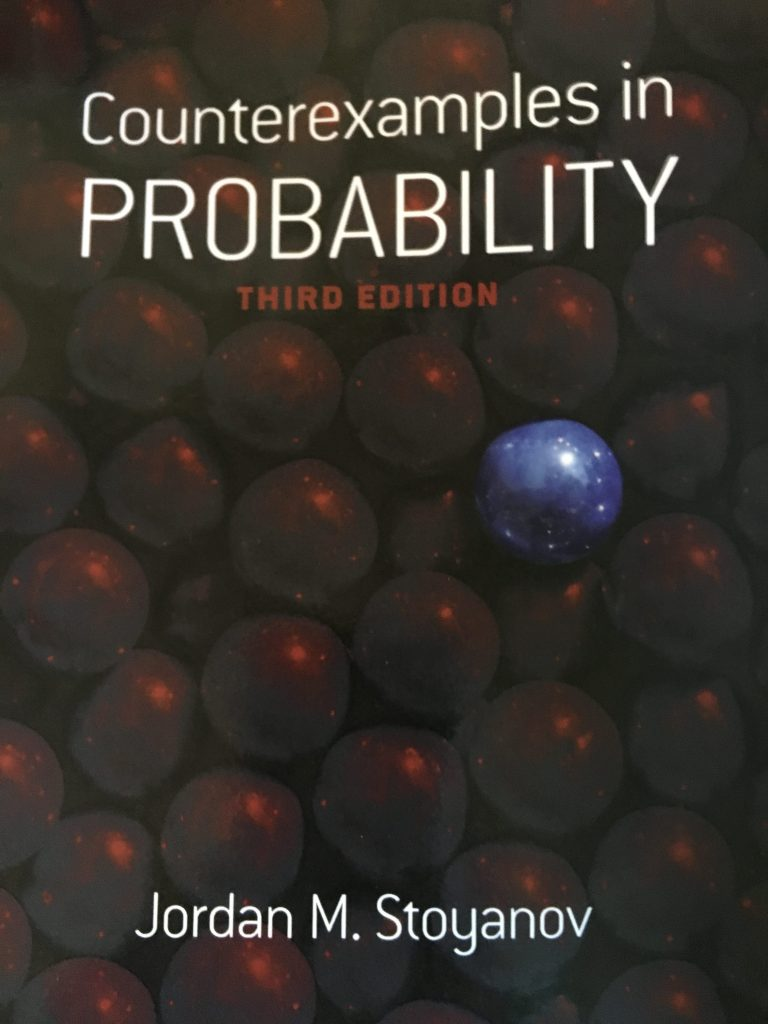## Book Review: Counterexamples in Probability, 3rd edition

### Author: J. Stoyanov

#### Reviewed by: R. Traylor## Ranking

All ranking is done on a scale of 1-5, with 5 as the best.
Attribute Rank
Difficulty 5
Proof Quality 3
Self-Study 2
Good for Teaching? 3
Quality of Exercises NA

## Topics Covered

Counterexamples in
• classes of random events
• probability measures
• independence of random events, notably
• pairwise independence doesn't imply mutual independence
• independent classes of random events can generate $\sigma$-fields which are not independent
• distribution functions, such as
• identically distributed random variables are not necessarily equivalent
• continuous 2D probability density with discontinuous marginal densities
• expectations and conditional expectations
• independence of random variables
• absolutely continuous random variables which are pairwise but not mutually independent
• characteristic and generating functions
• a characteristic function whose absolute value is not a characteristic function
• normal distribution studies
• moments
• other probability distributions
• properties that do not characterize various distributions
• convergence
• law of large numbers
• central limit theorem
• sequences of random variables that do not obey the CLT
• stochastic processes, martingales, and Poisson processes